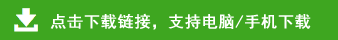# 小学一年级数学暑假作业全套

小学一年级数学暑假作业全套部分内容预览

3+48=       7+74=        43+9=        46+6=        65+7=

26+9=       17+6+8=      23+4+9=      62-50+7=     50+27-8=

50+50=      100-50=      20+40=       50+30=       50+20-30=

90-70+60=   16-6=        4+23=        33+6=        7+5=

1、一年级有98个同学去旅游。第一辆车只能坐40人，第二辆车能坐55人。还有多少人不能上车？

2、一年级有56人参加游园比赛。在第一轮比赛中，有28人走了，又来了37人参加第二轮比赛。现在有多少人参加游园比赛？

3、一双球鞋的价格是72元，一双布鞋的价格比一双球鞋的价格便宜了48元。一双布鞋的价格是多少元？